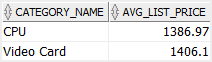# Oracle AVG

Summary: in this tutorial, you will learn how to use the Oracle `AVG()` function to calculate the average of a group.

## Oracle `AVG()` syntax

The Oracle `AVG()` function accepts a list of values and returns the average.

The following illustrates the syntax of the Oracle `AVG()` function:

```.wp-block-code {
border: 0;
}

.wp-block-code > div {
overflow: auto;
}

.shcb-language {
border: 0;
clip: rect(1px, 1px, 1px, 1px);
-webkit-clip-path: inset(50%);
clip-path: inset(50%);
height: 1px;
margin: -1px;
overflow: hidden;
position: absolute;
width: 1px;
word-wrap: normal;
word-break: normal;
}

.hljs {
box-sizing: border-box;
}

.hljs.shcb-code-table {
display: table;
width: 100%;
}

.hljs.shcb-code-table > .shcb-loc {
color: inherit;
display: table-row;
width: 100%;
}

.hljs.shcb-code-table .shcb-loc > span {
display: table-cell;
}

.wp-block-code code.hljs:not(.shcb-wrap-lines) {
white-space: pre;
}

.wp-block-code code.hljs.shcb-wrap-lines {
white-space: pre-wrap;
}

.hljs.shcb-line-numbers {
border-spacing: 0;
counter-reset: line;
}

.hljs.shcb-line-numbers > .shcb-loc {
counter-increment: line;
}

.hljs.shcb-line-numbers .shcb-loc > span {
}

.hljs.shcb-line-numbers .shcb-loc::before {
border-right: 1px solid #ddd;
content: counter(line);
display: table-cell;
text-align: right;
-webkit-user-select: none;
-moz-user-select: none;
-ms-user-select: none;
user-select: none;
white-space: nowrap;
width: 1%;
}```AVG([DISTINCT | ALL ] expression)
```Code language: SQL (Structured Query Language) (sql)```

The `AVG()` function can accept a clause which is either `DISTINCT` or `ALL`.

The `DISTINCT` clause instructs the function to ignore the duplicate values while the `ALL` clause causes the function to consider all the duplicate values.

For example, the average `DISTINCT` of 1, 1, 2, and 3 is (1 + 2 + 3 ) / 3 = 2, while the average `ALL` of 1, 1, 2, and 3 is (1 + 1 + 2 + 3) /4 = 1.75

The `AVG()` function ignores NULL values. For example, the average of 2, 4, and NULL is (2 + 4) /2 = 3.

## Oracle `AVG()` examples

We will use the `products` table in the sample database for the demonstration.

### A) Simple Oracle `AVG()` example

``````SELECT
ROUND(AVG( standard_cost ), 2) avg_std_cost
FROM
products;
```Code language: SQL (Structured Query Language) (sql)```

Notice that we used the `ROUND()` function to return the average standard cost rounded to 2 decimal places.

You can also use multiple `AVG()` functions in the same query. For example, the following statement calculates the averages of standard costs and list prices:

``````SELECT
ROUND(AVG( standard_cost ),2) avg_std_cost,
ROUND(AVG( list_price ), 2) avg_list_price
FROM
products;
```Code language: SQL (Structured Query Language) (sql)```

### B) Oracle `AGV()` with `DISTINCT` clause

The following statement calculates the average `DISTINCT` list prices:

``````SELECT
ROUND(
AVG( DISTINCT list_price ),
2
) avg_list_price
FROM
products;
```Code language: SQL (Structured Query Language) (sql)```

The result of the average `DISTINCT` is different from the average `ALL` above because some products have the same list prices.

### C) Oracle `AVG()` with `GROUP BY` clause

The following example calculates the average list price of products by category:

``````SELECT
category_id,
ROUND(
AVG( list_price ),
2
) avg_list_price
FROM
products
GROUP BY
category_id;
```Code language: SQL (Structured Query Language) (sql)```

In this example, the `GROUP BY` clause divides the products by category and then the `AVG()` function returns the average for each group.

To make the result more readable, you can also retrieve the category name by adding an `INNER JOIN` clause to the query above:

``````SELECT
category_name,
ROUND( AVG( list_price ),2 ) avg_list_price
FROM
products
INNER JOIN product_categories
USING(category_id)
GROUP BY
category_name
ORDER BY
category_name;
```Code language: SQL (Structured Query Language) (sql)```

### D) Oracle `AVG()` with `HAVING` clause

The following example returns the product categories whose average list prices are greater than 1000 specified by the `HAVING` clause:

``````SELECT
category_name,
ROUND(
AVG( list_price ),
2
) avg_list_price
FROM
products
INNER JOIN product_categories
USING(category_id)
GROUP BY
category_name
HAVING
AVG( list_price )> 1000
ORDER BY
category_name;
```Code language: SQL (Structured Query Language) (sql)```

###The following `HAVING` clause is used to filter groups of product categories.

``````HAVING
AVG( list_price )> 1000
```Code language: SQL (Structured Query Language) (sql)```

### E) Oracle `AVG()` with subquery

Consider the following example:

``````SELECT
ROUND( AVG( avg_list_price ), 2) avg_of_avg
FROM
(
SELECT
AVG( list_price ) avg_list_price
FROM
products
GROUP BY
category_id
);
```Code language: SQL (Structured Query Language) (sql)```

In this example:

• The subquery returns the average list prices by product category.
• The outer query returns the average of the average list prices per product category.

### F) Oracle `AVG()` with NULL values

Let’s create a new table named `tests` for the demonstration.

``````CREATE TABLE tests (
employee_id NUMBER PRIMARY KEY,
score NUMBER(3,1)
);

INSERT INTO tests(employee_id, score) VALUES(1, 95);
INSERT INTO tests(employee_id, score) VALUES(2, 70);
INSERT INTO tests(employee_id, score) VALUES(3, 60);
INSERT INTO tests(employee_id, score) VALUES(4, null);

SELECT * FROM tests;```Code language: SQL (Structured Query Language) (sql)```

The following statement calculates the average score of all employees:

``````SELECT
AVG( score )
FROM
tests;
```Code language: SQL (Structured Query Language) (sql)```

The result is:

As mentioned earlier, the `AVG()` function ignores NULL values.

### G) Oracle `AVG()` with `NVL()` function

If you want to treat the NULL value as zero for calculating the average, you can use `AVG()` function together with the `NVL()` function:

``````SELECT
AVG( NVL( score, 0 ))
FROM
tests;
```Code language: SQL (Structured Query Language) (sql)```

The `NVL()` function returns 0 if the score is null. Otherwise, it returns the score.

Here is the result:

In this tutorial, you have learned how to use the Oracle `AVG()` function to calculate the average of a group of values.